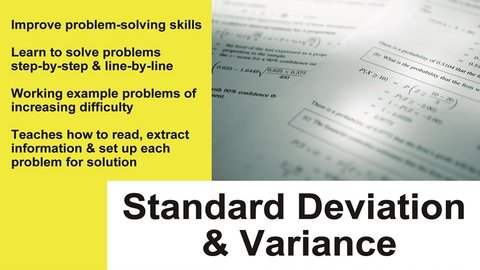# Standard Deviation and Variance Part of the Series: Probability and Statistics Tutor Series

##### TMW Media## Related videos

Mean & Standard Deviation of the Binomial Distribution
This program covers the important topic of the Mean and Standard Deviation of the Binomial Distribution in Probability and Statistics. We begin by discussing the Binomial Distribution and its mean. Next, we solve several problems that involve the Mean and Standard Deviation of this important distribution to give students practice…
Inverse Trigonometric Functions
This program covers the important topic of Inverse Trigonometric Functions in Calculus. We begin by discussing what an inverse trigonometric function is and why it is a central topic in Calculus. Next, we solve several practical calculus problems that give students practice with inverse trigonometric functions. The entire lesson is…
Derivatives of Inverse Trigonometric Functions
This program covers the important topic of Derivatives of Inverse Trigonometric Functions in Calculus. We begin by discussing what an inverse trigonometric function is, how to take its derivative, and why it is a central topic in Calculus. Next, we solve several practical calculus problems that give students practice with…
Hyperbolic Functions
This program covers the important topic of Hyperbolic Functions in Calculus. We begin by discussing what a Hyperbolic function is and why it is a central topic in Calculus. Next, we solve several practical calculus problems that give students practice with Hyperbolic functions. The entire lesson is taught by working…
Inverse Hyperbolic Functions
This program covers the important topic of Inverse Hyperbolic Functions in Calculus. We begin by discussing what an Inverse Hyperbolic function is and why it is a central topic in Calculus. Next, we solve several practical calculus problems that give students practice with Inverse Hyperbolic functions. The entire lesson is…
L'Hospital's Rule
This program covers the important topic of L'Hospital's Rule in Calculus. We begin by discussing what L'Hospital's Rule is, how it is used to take derivatives, and why it is a central topic in Calculus. Next, we solve several practical calculus problems that give students practice with L'Hospital's Rule. The…
Trigonometric Integrals
This program covers the important topic of Trigonometric Integrals in Calculus. We begin by discussing what Trigonometric Integrals are and why it is a central topic in Calculus. Next, we solve several practical calculus problems that give students practice with Trigonometric Integrals. The entire lesson is taught by working example…
Integration By Partial Fractions
This program covers the important topic of Integration by Partial Fractions in Calculus. We begin by discussing what a Partial Fraction is, how it can be used to solve integrals, and why it is a central topic in Calculus. Next, we solve several practical calculus problems that give students practice…
Arc Length
This program covers the important topic of Arc Length in Calculus. We begin by discussing what Arc Length is and why it is a central topic in Calculus. Next, we solve several practical calculus problems that give students practice with Arc Length. The entire lesson is taught by working example…
Area of a Surface of Revolution
This program covers the important topic of Area of a Surface of Revolution in Calculus. We begin by discussing what a Surface of Revolution is and why it is a central topic in Calculus. Next, we solve several practical calculus problems that give students practice with finding the Area of…
Parametric Equations
This program covers the important topic of Parametric Equations in Calculus. We begin by discussing what a Parametric Equation is and why it is a central topic in Calculus. Next, we solve several practical calculus problems that give students practice with Parametric Equations. The entire lesson is taught by working…
Arc Length In Parametric Equations
This program covers the important topic of calculating Arc Length with Parametric Equations in Calculus. We begin by discussing what an Arc Length is in the context of Parametric Equations is and why it is a central topic in Calculus. Next, we solve several practical calculus problems that give students…